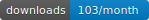# sim1000Gstatistics

R package for simulating genetic marker data.

## Installation

The most stable version can be found at CRAN:

``install.packages("sim1000G")``

## Quickstart

The following script will generate variants in the region of the example vcf file for 200 unrelated individuals:

``````library(sim1000G)

# Read the example file included in sim1000G

examples_dir = system.file("examples", package = "sim1000G")
vcf_file = file.path(examples_dir,"region.vcf.gz")

# Alternatively provide a vcf file here:
# vcf_file = "~/fs/tmp/sim4/pop1/region-chr4-312-GABRB1.vcf.gz"

vcf = readVCF( vcf_file, maxNumberOfVariants = 600 , min_maf = 0.01, max_maf = 1)

startSimulation(vcf, totalNumberOfIndividuals = 1000)
ids = generateUnrelatedIndividuals(200)

genotype = retrieveGenotypes(ids)

``````

With the genotypes we can compare the allele frequencies with the ones in the original vcf file:

``````
# Compare MAF of simulataed data and vcf
plot( apply(genotype,2,mean)/2 ,  apply(vcf\$gt1+vcf\$gt2,1,mean)/2 )
abline(0,1,lty=1,lwd=9,col=rgb(0,0,1,0.3))``````

An image showing the generated genotypes:

``````
# show the genotypes as an image

gplots::heatmap.2(genotype,col=c("white","orange","red"),Colv=F, trace="none")``````

We can also compute the correlation between the markers and show an LD plot of the region:

``````
# LD plot of region

gplots::heatmap.2( cor(genotype)^2 , trace="none", col=rev(heat.colors(200)) ,Rowv=F,Colv=F )

``````

## Documenation

A more detailed documentation and code examples can be found at:

SimulatingFamilyData